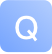# 如何在插入排序中将数据配对在一起 -问答-阿里云开发者社区-阿里云## 如何在插入排序中将数据配对在一起

2020-04-11 15:53:03 887 1

for (int i = 1; i <= count; i++) { for (int j = 0; j < count - 1; j++) { if (email [j].compareToIgnoreCase (email [j + 1]) > 0) { temp1 = email [j]; email [j] = email [j + 1]; email [j + 1] = temp1;

``````             temp2 = fname [j];
fname [j] = fname [j + 1];
fname [j + 1] = temp2;

temp3 = lname [j];
lname [j] = lname [j + 1];
lname [j + 1] = temp3;

temp4 = city [j];
city [j] = city [j + 1];
city [j + 1] = temp4;

temp5 = age [j];
age [j] = age [j + 1];
age [j + 1] = temp5;
}
}
}
``````

}

for (int i = 1; i < count; i++) { String current = fname [i]; int j = i - 1;

``````    while (j >= 0 && current.compareToIgnoreCase (fname [j]) < 0)
{
fname [j + 1] = fname [j];
j--;
}
fname [j + 1] = current;
}
``````取消 提交回答

• 七天一失眠
2020-04-11 15:53:13

您通过气泡比较排序，然后通过邮件比较，然后是其他字段，但是插入仅比较名称，为什么？也许您不应该在插入内容中与其他内容进行比较，以便于订购

{for (int i = 1; i < count; i++) String current = fname [i]; int j = i - 1;

``````while ((j >= 0 && current.compareToIgnoreCase (fname [j]) < 0) and j>=0 && current.compareToIgnoreCase (email[j]....)
{
fname [j + 1] = fname [j];
j--;
}
fname [j + 1] = current;
``````

}

答案来源：stackoverflow

0 0

0

100

JS零基础入门教程（上册）